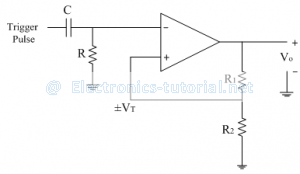Home > analog integrated circuits > multivibrators > bistable multivibrators

# Bistable multivibrators

In this circuit, both the states at the output (+Vsat and -Vsat) are stable states. i.e. the circuit remains in the same state till the external input is applied. If we want to change the output state a triggering pulse is applied. Now the state obtained after the pulse is applied is a permanent stable state. If we want to change the state again, we have to apply a triggering pulse. Thus by only application of trigger pulse, output changes its state. Thus to get the original state back, two triggering pulses need to be applied. So the frequency of the output is half of the triggering pulse frequency. Thus it is also called as divide by 2 circuits. The examples of such divide by 2 networks are binary counters, flip-flops etc. The following figure shows the bistable multivibrator using op-amp.Operation:
Let us assume that the output is in positive saturation i.e. +Vsat. Thus the threshold voltage(at non-inverting terminal) is also positive and is given as
V^+=VT=R_2/(R1+R2 )(Vsat)
Initially no input signal is applied at inverting terminal of op-amp. Thus the voltage at inverting terminal is V^-=0
In order to change the state of the output from +Vsat to –Vsat, a positive pulse is applied at inverting terminal of op-amp. At instant 't1' a trigger pulse is applied as shown in the waveforms below.To change the state of the output, the magnitude of the trigger pulse (i.e. voltage at inverting terminal) should be greater than the positive threshold +VT.
∴V^->R2/(R1+R2 )(Vsat)
After the application of the pulse with required amplitude, the output state is changed from +Vsat to –Vsat.
Now in the stable state(-Vsat), the threshold voltage(at non-inverting terminal) is also negative and is given as
V^+=VT=R2/(R1+R2 )(-Vsat)
Again in order to change the state from –Vsat to +Vsat, a negative pulse is applied at inverting terminal of op-amp. At instant 't2' a trigger pulse is applied as shown in the waveforms. To change the state of the output, the magnitude of the trigger pulse (i.e. voltage at inverting terminal) should be greater than the negative threshold.
∴V^->R2/(R1+R2 )(-Vsat)